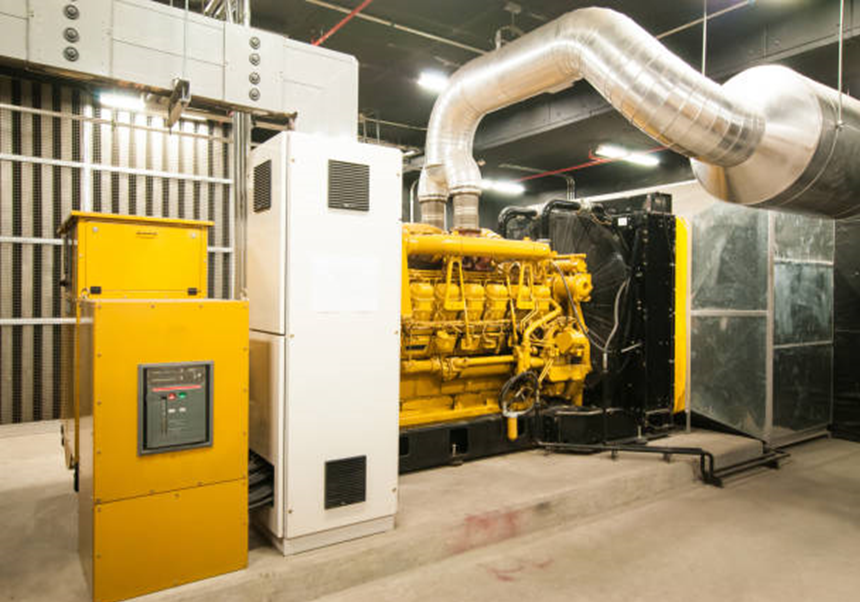# Generator Noise Calculator

This generator noise calculator can be used to calculate the approximate sound power level of an industrial generator. In Engineering Noise Control by Bies and Hansen, they have developed methods to estimate Sound Power (Lw) or Sound Pressure (Lp) noise levels for various industrial equipment items. The tool below allows for calculation of the Lw of Industrial Generators (excluding the driver) based on the calculations in Engineering Noise Control.In order to derive the sound pressure level from the sound power level, our sound pressure – sound power converter tool can be used. Make note of the calculated sound power level calculated here – you will need it to estimate the sound pressure level.